# Important Questions CBSE Class 9 Maths Chapter 3-Coordinate Geometry

Important questions for CBSE Class 9 Maths chapter 3 coordinate geometry are available with solutions here, by our subject experts. Students preparing for 9th standard final exams can practice these questions to score good marks. All the problems and solutions here are as per CBSE syllabus and taken from NCERT book.

To revise all the chapters go to important questions for CBSE Class 9 Maths at BYJU’S. Also, practice extra questions provided in each chapter to have better preparation for Maths subject exam.

In coordinate geometry chapter, students will learn to locate the points in an XY plane or in a cartesian system. This concept is helpful to find the location of an object in a particular place. Its major application is locating the places in a map or globe. Let us solve questions based on this concept and understand it well.

Also Check:

## Important Questions & Solutions for CBSE Class 9 Chapter 3 (Coordinate Geometry)

1. Write the coordinates of each of the points P, Q, R, S, T and O from the figure given.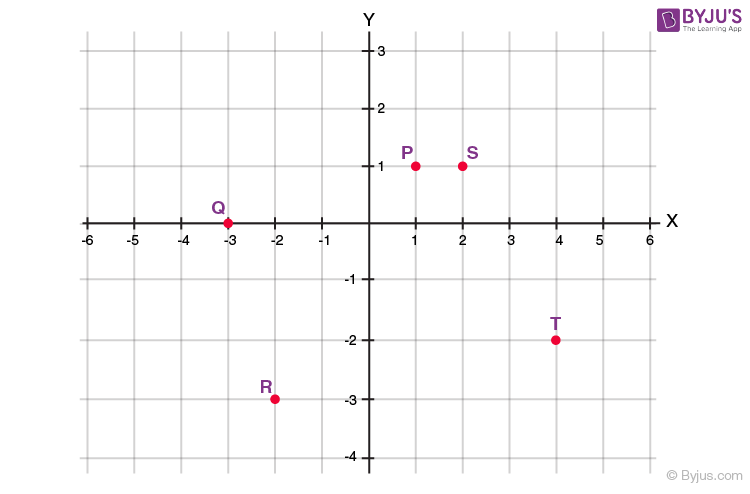Solution:

The coordinates of the points P, Q, R, S, T and O are as follows:

P = (1, 1)

Q = (-3, 0)

R = (-2, -3)

S = (2, 1)

T = (4, -2)

O = (0, 0)

Q.2: Plot the following points and check whether they are collinear or not:

(i) (1, 3), (– 1, – 1), (– 2, – 3)

(ii) (1, 1), (2, – 3), (– 1, – 2)

(iii) (0, 0), (2, 2), (5, 5)

Solution:

(i)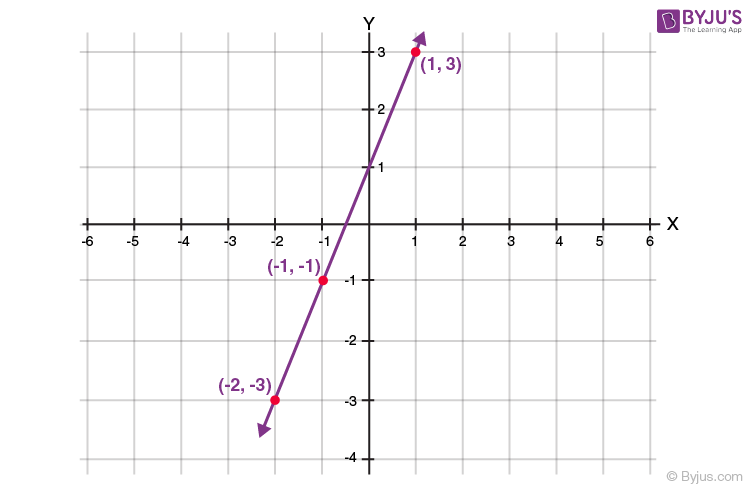The points (1, 3), (– 1, – 1), (– 2, – 3) lie in a straight line,

Hence, the points are collinear.

(ii)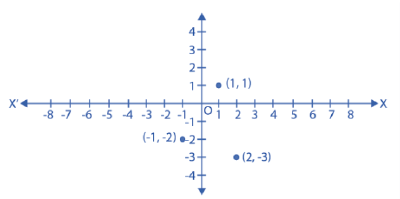The points (1, 1), (2, – 3), (– 1, – 2) do not lie in a straight line,

Hence, the points are not collinear.

(iii)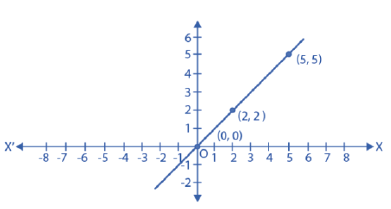The points (0, 0), (2, 2), (5, 5) lie in a straight line,

Hence, the points are collinear.

Q.3: Without plotting the points indicate the quadrant in which they will lie, if

(i) the ordinate is 5 and abscissa is – 3

(ii) the abscissa is – 5 and ordinate is – 3

(iii) the abscissa is – 5 and ordinate is 3

(iv) the ordinate is 5 and abscissa is 3

Solution:

(i) The point is (-3,5).

Hence, the point lies in the II quadrant.

(ii) The point is (-5,-3).

Hence, the point lies in the III quadrant.

(iii) The point is (-5,3).

Hence, the point lies in the II quadrant.

(iv) The point is (3,5).

Hence, the point lies in the I quadrant.

Q.4: See figure and write the following:

1. The coordinates of B.
2. The coordinates of C.
3. The point identified by the coordinates (–3, –5).
4. The point identified by the coordinates (2, – 4).
5. The abscissa of the point D.
6. The ordinate of the point H.
7. The coordinates of the point L.
8. The coordinates of the point M.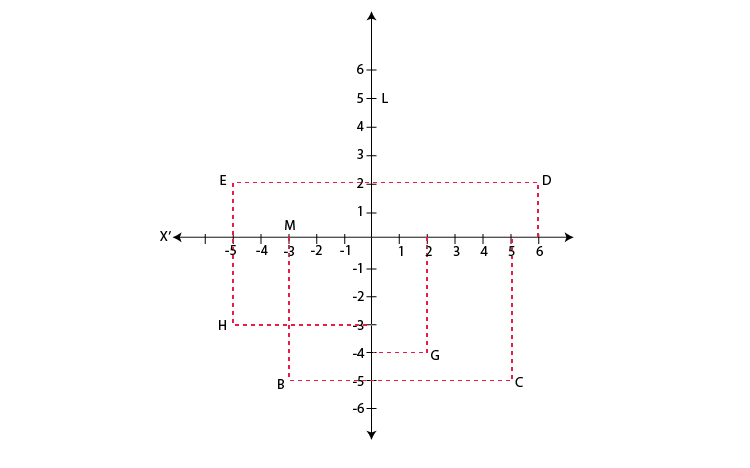Solution:

1. The co-ordinates of B is (-3, -5).
2. The co-ordinates of C is (5, −5).
3. The point identified by the coordinates (−3, −5) is B.
4. The point identified by the coordinates (2, −4) is G.
5. Abscissa means x co-ordinate of point D. So, the abscissa of the point D is 6.
6. Ordinate means y coordinate of point H. So, the ordinate of point H is -3.
7. The coordinates of the point L is (0, 5).
8. The coordinates of the point M is (−3, 0).

Q.5: Plot the points (x, y) given in the following table on the plane, choosing suitable units of distance on the axes.

 x -2 -1 0 1 3 y 8 7 -1.25 3 -1

Solution:

The points to plotted on the(x,y) are:

1. (-2,8)
2. (-1,7)
3. (0,-1.25)
4. (1,3)
5. (3,-1)

On the graph mark X-axis and Y-axis. Mark the meeting point as O.

Now, Let 1 unit = 1 cm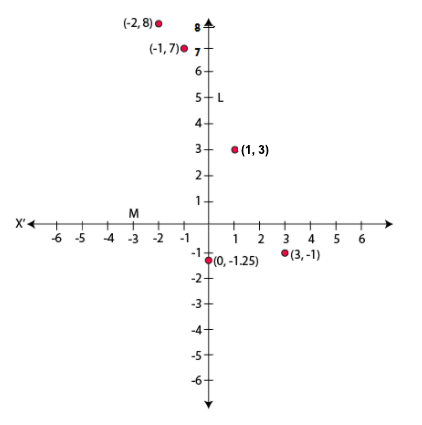Q.6: Write the answer to each of the following questions:

(i) What is the name of the horizontal and the vertical lines drawn to determine the position of any point in the Cartesian plane?

(ii) What is the name of each part of the plane formed by these two lines?

(iii) Write the name of the point where these two lines intersect

Solution:

(i) The name of horizontal and vertical lines drawn to determine the position of any point in the Cartesian plane is x-axis and y-axis respectively.

(ii) The name of each part of the plane formed by these two lines x-axis and the y-axis is called quadrants.

(iii) The point where these two lines intersect is called the origin

### Extra Questions For Class 9th Maths Chapter 3

1. Points A (5, 3), B (–2, 3) and D (5, –4) are three vertices of a square ABCD. Plot these points on a graph paper and hence find the coordinates of the vertex C.

2. Write the coordinates of the vertices of a rectangle whose length and breadth are 5 and 3 units respectively, one vertex is at the origin, the longer side lies on the x-axis, and one of the vertices lies in the third quadrant.

3. Plot the points (x, y) given by the following table:

 x 2 4 -3 -2 y 4 2 0 5

4. Plot the following points and write the name of the figure obtained by joining them in order:

P(– 3, 2), Q (– 7, – 3), R (6, – 3), S (2, 2)

5. Locate the points (5, 0), (0, 5), (2, 5), (5, 2), (–3, 5), (–3, –5), (5, –3) and (6, 1) in the Cartesian plane.

6. Solve these following equations:

(i) 3x + 3 = 15

(ii) 2y + 7 =19

1. nice but 4 marks or 3 marks questio should also be added

1. lavanya
1. Thank you these will help me in my exams

2. Thank u for the important question

This is soo helpful for my exams! Tysm!!!

4. Amal James

very good questions

5. nishant chauhan

these questions are very nice . They are very helpful for me.
THANKS FOR GIVING SUCH QUESTIONS

6. mamta rathore

thankyuu soo much byjus

7. Please sir provide questions in hindi medium also

8. LAKSHYA JAIN

THANKS THESE QUESTIONS VERY INTERESTING AND HELPFUL.

9. Ishan Gupta

Thanks for these questions